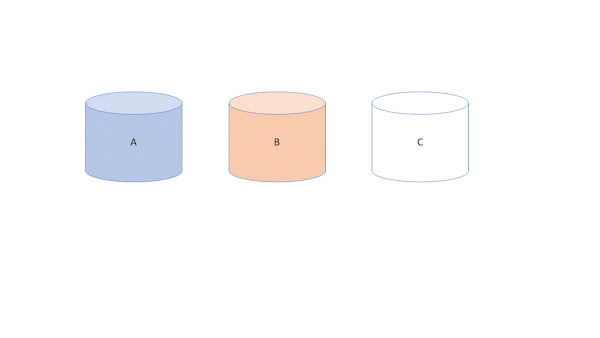Select Page

# Swapping of Two Numbers in ABAP

by | Jun 3, 2018 | ABAP Programs

Home » SAP » ABAP » ABAP Programs » Swapping of Two Numbers in ABAP

Preface – This post is part of the ABAP Programs series.

Swapping of Two Numbers in ABAP  is a way to interchange the value of two variables with each other. It is rarely used in coding but it is an important topic for interviews. In this article we will cover both: swapping using third variable and swapping without using third variable in ABAP.

## Introduction

Everyone is aware what a swap is. A Swap is a way to exchange the value of two variables with each other. There are many ways to do swapping in ABAP:

## Swapping using third variable:## Swapping of Two Numbers Image Illustration

Here we save the value of 1st variable into a new 3rd variable. Now the 1st variable saves the value of 2nd variable and the 2nd variable saves the value of 3rd variable. In this way the value is exchanged.

```*------Do not copy this code, this is just for illustration-------*
Data: lv_var1, lv_var2, lv_var3. "Here we have 3 variables
lv_var1 = 2. "Assinging values to variables
lv_var2 = 5.
* swapping code
lv_var3 = lv_var1. "Saving value of var 1 in var 3
lv_var1 = lv_var2. "Saving value of var 2 in var 1
lv_var2 = lv_var3. "Saving value of var 3 in var 1
*** Now the output will show lv_var1 = 5 and lv_var2  = 2```

## Swapping without using third variable:

This can be achieved using either addition-subtraction or multiplication-division methods. We will show how in below illustration code:

```*------Do not copy this code, this is just for illustration-------*
Data: lv_var1, lv_var2. "Here we have 2 variables
lv_var1 = 2. "Assinging values to variables
lv_var2 = 5.
* swapping code
lv_var1 = lv_var1 + lv_var2 . "Now lv_var1 = 2+5 = 7.
lv_var2 = lv_var1 - lv_var2. "Now lv_var2 = 7-5 = 2.
lv_var1 = lv_var1 - lv_var2. "Now lv_var1 = 7-2 = 5.
*** Now the output will show lv_var1 = 5 and lv_var2  = 2```

Similarly using multiplication-division, we will achieve the same.

Now the ABAP program to achieve the swap is shown below:

```PARAMETERS : lv_num1(4) type i,
lv_num2(4) type i.
lv_num1 = lv_num1 + lv_num2.
lv_num2 = lv_num1 - lv_num2.
lv_num1 = lv_num1 - lv_num2.
Write: 'Swapped Values are :', lv_num1, lv_num2.
```

## Author

•A Full Stack Developer with 10+ years of experience in technical content creation.

•